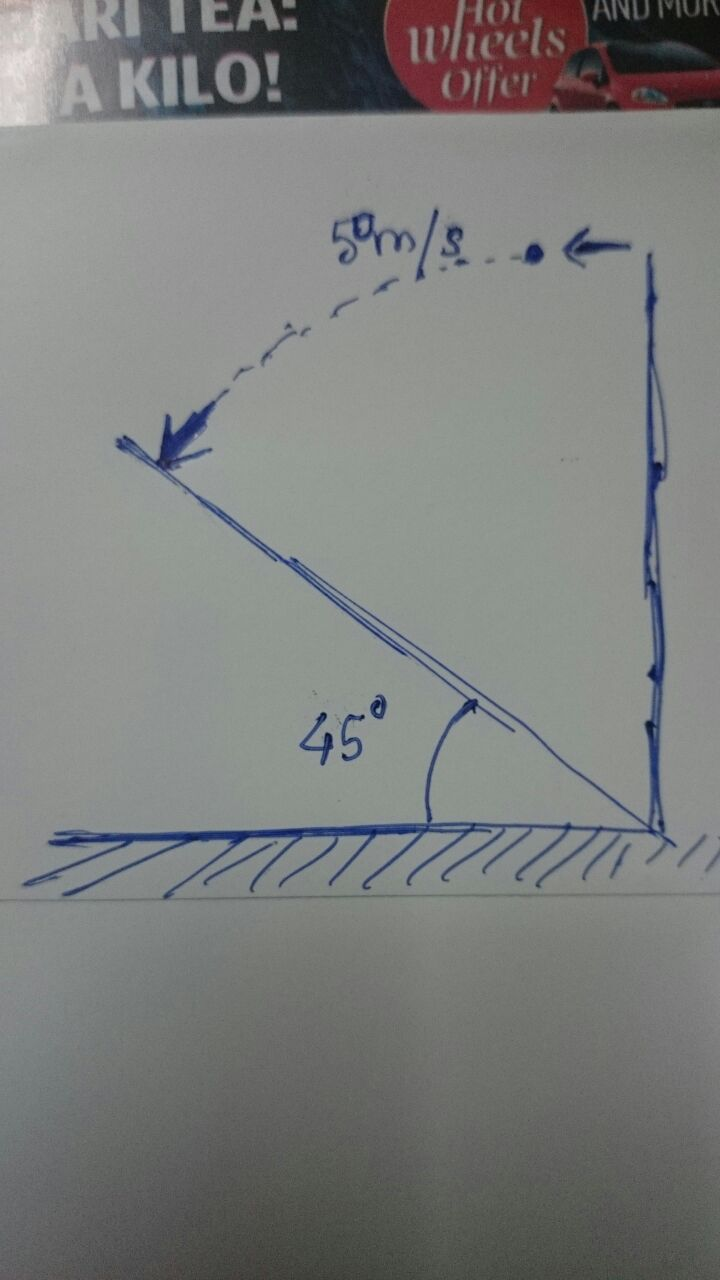# An Original ProjectileA ball is thrown horizontally from top of a tall tower at 50 m/s. The ground below is a uniform slope upwards of 45 degrees. The direction of the ball when it hits the slope is exactly perpendicular to the slope.

After how many seconds did the ball hit the ground ?

Take g = 9.8 m/s^2

×